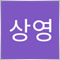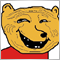# I have curious about rates_total/prev_calculated90

Hello, I'm beginner.

I have some curious.

I run indicator.

```int OnCalculate(const int rates_total,
const int prev_calculated,
const datetime &time[],
const double &open[],
const double &high[],
const double &low[],
const double &close[],
const long &tick_volume[],
const long &volume[],
{
Comment( "rates_total : ",rates_total,"\n",
"prev_calculated : ",prev_calculated,"\n");```

I thought rates_total / prev_calculated similar candle.

but now I don't think it might be.

the reasone why

M1 total is 66617,

M5 total is 65002,

M15 total is 65000

M30 total is 65001

H1 total is 65004

H4 total is 38127

D1 total is 12858

W1 total is 2422

MN total is 570.

I feel that is not relevant.

What is mean rates_total and prev_calculated?1079

Returned rates_total from previous round of OnCalculate becomes prev_calculated in current round. They mostly equal except when a new bar appears (rates_total=prev_calculated+1) or chart is refreshed with a new history (prev_calculate=0 set by terminal).90

kypa:
Returned rates_total from previous round of OnCalculate becomes prev_calculated in current round. They mostly equal except when a new bar appears (rates_total=prev_calculated+1) or chart is refreshed with a new history (prev_calculate=0 set by terminal).

Thank you!

I have more question .

I think if D1 is 12858, H4 is 12858*3 (38547). But H4 is 38127. Why...?

Thank you kypa !1079

That's probably all the history available. Pre-holiday sessions may end earlier (less H4 bars) and still be recorded as daily bars.

In MT5 history is recorded in 1 minute bars, all other timeframes are recalculated from it. If there is no tick through the minute no bar is made.

You can limit the bars from tools/settings/charts.90

kypa:

That's probably all the history available. Pre-holiday sessions may end earlier (less H4 bars) and still be recorded as daily bars.

In MT5 history is recorded in 1 minute bars, all other timeframes are recalculated from it. If there is no tick through the minute no bar is made.

You can limit the bars from tools/settings/charts.

Ah! I understand ! Thank you very much kypa!